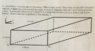# Hall Effect

## Homework Statement

A metal strip 6.5cm long, 0.88cm wide, and 0.76cm thick moves with constant velocity, magnitude v along positive y-axis, through a magnetic field, B=1.2mT along positive z-axis. A potential difference of 3.9x10^-6V is measured between points x1 and x2 (as shown in halleffect.jpg). Determine the magnitude of the velocity, v.

Vh=BVW

## The Attempt at a Solution

info given:
length=6.5cm
width=0.88cm
thickness=0.76cm
B=1.2mT
Vh=3.9x10^-6V

Vh=BVW
V=(Vh)/BW→(3.9x1-^-6V)/((1.2x10^-3T)(0.88x10^-2m)→0.369m/s

Im wondering if I am missing something, this problem seems too easy.

Any insight would be appreciated!

Thanks

#### Attachments

•Halleffect.JPG
39.3 KB · Views: 196

•